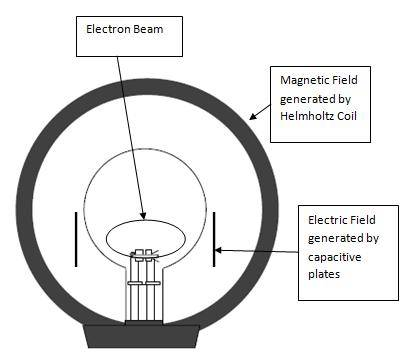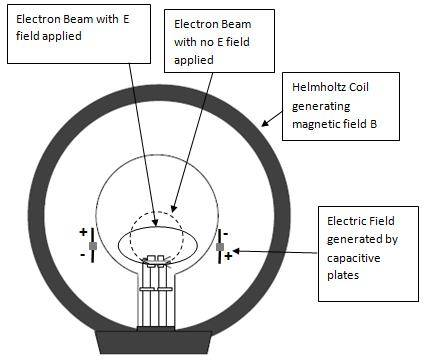# Calculation of an Electron Beam path when subjected to magnetic and electric fields

HI all, I have been trying to calculate the displacement of an electron when subjected electric and magnetic field, please see the pic below:I anticipate that the electron will form an ellipse shape. Basically I would like to know how to calculate the major and minor axis of the ellipse.

I know how to calculate the electrostatic deflection of an electron beam, and I know how to calculate the magnetic deflection of an electron beam. I am guessing you got to combine these two ideas, but I cant figure out how. Hopefully there are some more intelligent people in here than me.

I thank you in advance

Jon

## Answers and Replies

look up drift of charged particle in electric and magnetic field. guiding center may also be a useful search term.

I forgot to mention that as well as magnetic and electric fields there will be a accelerating voltage on the anode.

I cannot find much, electromagnetics is not my strongest subject

You start with lorentz force law. Your setup has created an electric field perpendicular to the magnetic field. You can create a coordinate system to align one coordinate to magnetic field, and one to electric field. It takes some knowledge of solving differential equations to get the solution, but it is possible.

What you will find is that the electron does not get back to where it started. Its path does not form an ellipse. The loop it forms without the electric field in effect drifts perpendicular to the electric field: http://en.wikipedia.org/wiki/Guiding_center

What you will find is that the electron does not get back to where it started. Its path does not form an ellipse. The loop it forms without the electric field in effect drifts perpendicular to the electric field: http://en.wikipedia.org/wiki/Guiding_center

I have seen this in the videos demonstrating the effect of the helmholtz coil on an electron beam. The electron beam does not finish at its starting place. Instead it finishes inside the circle - closer to the centre of the circle, in other words it travels in a spiral. But what I meant is what will happen to the shape of this when an electric field is applied, will it cause the shape to go more elliptical ?

Last edited:

I have seen this in the videos demonstrating the effect of the helmholtz coil on an electron beam. The electron beam does not finish at its starting place. Instead it finishes inside the circle - closer to the centre of the circle, in other words it travels in a spiral.......
Actually not. The Lorentz v x B force is always perpendicular to the velocity, so the electron beam goes in a circle. There is no force parallel to the velocity, so no work is done and the beam does not lose energy. See thumbnail photo of electron beam in a Helmholtz coil.

Bob S

#### Attachments

Does anyone to know how to actually calculate this?

To calculate the orbit of a charged particle in a magnetic field, we equate the Lorentz force and the centripital force (where e = charge, v = velocity, B = magnetic field, m = rest mass, and R = radius of orbit).:

evB = mv2/R

or BR = mv/e, where mv is momentum

At low energies we can write mv = [2mT]½ where T is kinetic energy, so we have

BR = [2mT]½/e Tesla-meters

We can do all of this in eV (electron volts and volts) as follows (where c is speed of light)

BR = [2mc2T]½/ec

Example 1) So for electrons with mc2 = 511,000 electron volts, we have

BR = [2·511,000·T]½/c where c=3 x 108 meters/sec

where I have divided electron volts by e to get volts.
So for a T = 300 volt electron beam,

BR = [2·511,000·300]½/3 x 108 = 5.84 x 10-5 Tesla-meters.

So for a field of 10 Gauss (0.001 Tesla), the orbit radius is 0.0583 meters (5.83 cm).

At high (relativistic) energies we use pc = [(mc2+T)2 - (mc2)2]½ = βγ mc2

where mc2 is particle rest mass and pc is momentum in pc (energy) units.

Example 2) For LHC at 7 GeV, mc2 =938.3 MeV and γ = 7460, so

BR = 7 x 1012/3 x 108 = 23,333 Tesla meters.

BR is sometimes called beam rigidity.

So for R ≈ 4200 meters, B ≈ 5.6 Tesla (these are approximate values). Actual values in LHC arcs at 7 TeV are B = 8.33 Tesla and R = 2802 meters.

This doesn't include the electric field, however.

Bob S

Last edited:

Including the electric field is harder. I will just write down the major steps of one way here.

$$\vec{F} = q(\vec{E}+\vec{v}\times \vec{B})$$

decompose with B || z, E || y.

$$\frac{d v_x}{dt} = \frac{q}{m}(v_y B)$$

$$\frac{d v_y}{dt} = \frac{q}{m}(E - v_x B)$$

$$\frac{d v_z}{dt} = 0$$

By taking the derivative with time on one and inserting into the other one can decompose the two coupled equations.

also setting frequency to qB/m, the cyclotron frequency

$$\omega = \frac{qB}{m}$$

$$\frac{d^2 v_x}{dt^2} = \omega \frac{d v_y}{dt} = \omega \frac{q}{m}E -\omega^2 v_x$$

re-arrange and it appears as a harmonic oscillator with a "forcing" function:

$$\frac{d^2 v_x}{dt^2} + \omega^2 v_x = \omega \frac{q}{m}E$$

the y component is similar with no "forcing".

$$\frac{d^2 v_y}{dt^2} + \omega^2 v_y = 0$$

Solving the homogeneous terms gives you solution to harmonic oscillator solution for velocity in both x and y. Solving the particular on for the x gives a constant additional velocity in x direction. That is the drift velocity. You can then take the time integral to find the position as a function of time.

[edit: and the general solutions]

$$v_x = A*sin(\omega t) + B*cos(\omega t) + \frac{E}{B}$$
$$v_y = C*sin(\omega t) + D*cos(\omega t)$$

A, B, C, D come from initial conditions. Also, from the original equation you will see that it must be that D = A and C = -B. So...

$$v_x = v_{y0}*sin(\omega t) + (v_{x0} - \frac{E}{B})*cos(\omega t) + \frac{E}{B}$$
$$v_y = -(v_{x0} - \frac{E}{B})}*sin(\omega t) + v_{y0}*cos(\omega t)$$

Last edited:

Thanks Bob S and kcdodd! I am studying your work now. Thanks again...

Bob_S where why do you equate the centripetal force to the Lorentz force. The centripetal force is the force created by the attraction of the anode and the Lorentz for the force created by the magnetic field acting on the electron, shouldn't these be added together?

To calculate the orbit of a charged particle in a magnetic field, we equate the Lorentz force and the centripital force (where e = charge, v = velocity, B = magnetic field, m = rest mass, and R = radius of orbit).:

evB = mv2/R

KC where did you pull these equations from?

$$v_x = A*sin(\omega t) + B*cos(\omega t) + \frac{E}{B}$$
$$v_y = C*sin(\omega t) + D*cos(\omega t)$$

A, B, C, D come from initial conditions. Also, from the original equation you will see that it must be that D = A and C = -B. So...

Thanks again guys for your help....I now understand why the beam will be drifting in a moving spiral. is that correct?

However I do not want to create a moving spiral. I want to create an stationary ellipse using magnetic and electric fields. I have come to the conclusion that in order to do this you need to use the following configuration:Any ideas on how to calculate this? :yuck:

Eternally grateful

Jon

Last edited:

Does any one have any idea of how to solve the above configuration ?

Does any one have any idea of how to solve the above configuration ?
Here is the solution with the electron source (filament) in the center of the positive anode structure, which is the same as a magnetron with a radial electric field and an axial magnetic field. See
http://books.google.com/books?id=ZM...k_result&ct=result&resnum=6&ved=0CDAQ6AEwBTge

Study equations 9.80 through 9.92. Are you proposing opposite polarity dc voltages on each end of each electrode plate?

Bob S

Thank you Bob, much appreciated, I will study the equations this weekend. Yes I am proposing opposite polarity DC on each end of the electrode plates, the little grey block separating them will be a high voltage insulator. You need this configuration in order to form an ellipse, and that is what I need an ellipse.

Are you proposing opposite polarity dc voltages on each end of each electrode plate?

Bob S

Yeh unfortunately the link is does not fully answer my question. The beam will still drift.

Yeh unfortunately the link is does not fully answer my question. The beam will still drift.
Your proposal to have opposite polarity on each end of each electrode may solve the E x B drift problem. The direction of the drift is in the plane of your illustration (perpendicular to B), and in the vertical direction (perpendicular to E). I have not convinced myself yet that the particle moves toward or away from the center though. The orbit certainly will not be an ellipse.

Bob S

I think it will be ellipse, if you think about it the electron picks up no net electron volts.
At the major axis extremity, the electron velocity will be at its maximum. At the minor axis extremity the electron will be at its lowest velocity but will be at its maximum acceleration.
Why do think, the shape wont be an ellipse?

Your proposal to have opposite polarity on each end of each electrode may solve the E x B drift problem. The direction of the drift is in the plane of your illustration (perpendicular to B), and in the vertical direction (perpendicular to E). I have not convinced myself yet that the particle moves toward or away from the center though. The orbit certainly will not be an ellipse.

Bob S